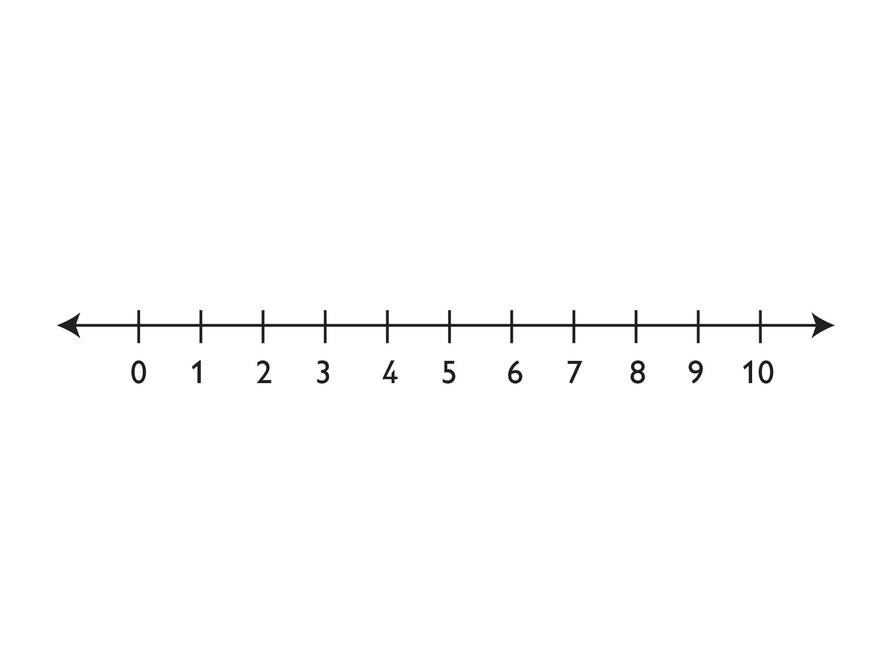# On the Number Line

Look at the portion of the number line shown. Use it to help you do problems 1–3. Either copy the number line to your Notebook, or draw your own number line to show your answers.

1. Find a value for j that makes $\frac{4}{j}<1$ true.
• Mark this value of on a number line.
• Is your value the only one possible? If so, say why. If not, describe the set of possible values.

2.  Find a value for k that makes $\frac{4}{k}>1$ true.

• Mark this value of on a number line.
• Is your value the only one possible? If so, say why. If not, describe the set of possible values

3. Find a value for that makes $\frac{4}{m}=1$ true.

• Mark this value of on a number line.
• Is your value the only one possible? If so, say why. If not, describe the set of possible values.